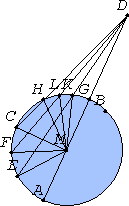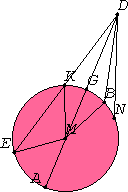# Proposition 8

If a point is taken outside a circle and from the point straight lines are drawn through to the circle, one of which is through the center and the others are drawn at random, then, of the straight lines which fall on the concave circumference, that through the center is greatest, while of the rest the nearer to that through the center is always greater than the more remote, but, of the straight lines falling on the convex circumference, that between the point and the diameter is least, while of the rest the nearer to the least is always less than the more remote; and only two equal straight lines fall on the circle from the point, one on each side of the least.

Let ABC be a circle, and let a point D be taken outside ABC. Let straight lines DA, DE, DF, and DC be drawn through from D, and let DA be drawn through the center.I say that, of the straight lines falling on the concave circumference AEFC, the straight line DA through the center is greatest, while DE is greater than DF, and DF greater than DC. But, of the straight lines falling on the convex circumference HLKG, the straight line DG between the point and the diameter AG is least, and the nearer to the least DG is always less than the more remote, namely DK is less than DL, and DL is less than DH.

III.1

Take the center M of the circle ABC. Join ME, MF, MC, MK, ML, and MH.

Then, since AM equals EM, add MD to each, therefore AD equals the sum of EM and MD.

I.20

But the sum of EM and MD is greater than ED, therefore AD is also greater than ED.

I.24

Again, since ME equals MF, and MD is common, therefore EM and MD equal FM and MD, and the angle EMD is greater than the angle FMD, therefore the base ED is greater than the base FD.

Similarly we can prove that FD is greater than CD. Therefore DA is greatest, while DE is greater than DF, and DF is greater than DC.

I.20

Next, since the sum of MK and KD is greater than MD, and MG equals MK, therefore the remainder KD is greater than the remainder GD, so that GD is less than KD.

I.21

And, since on MD, one of the sides of the triangle MLD, two straight lines MK and KD are constructed meeting within the triangle, therefore the sum of MK and KD is less than the sum of ML and LD. And MK equals ML, therefore the remainder DK is less than the remainder DL.

Similarly we can prove that DL is also less than DH. Therefore DG is least, while DK is less than DL, and DL is less than DH.I say also that only two equal straight lines will fall from the point D on the circle, one on each side of the least DG.

I.23

Construct the angle DMB equal to the angle KMD on the straight line MD and at the point M on it. Join DB.

I.4

Then, since MK equals MB, and MD is common, the two sides KM and MD equal the two sides BM and MD respectively, and the angle KMD equals the angle BMD, therefore the base DK equals the base DB.

I say that no other straight line equal to the straight line DK falls on the circle from the point D.

Above

For, if possible, let a straight line so fall, and let it be DN. Then, since DK equals DN, and DK equals DB, DB also equals DN, that is, the nearer to the least DG equal to the more remote, which was proved impossible.

Therefore no more than two equal straight lines fall on the circle ABC from the point D, one on each side of DG the least.

Therefore if a point is taken outside a circle and from the point straight lines are drawn through to the circle, one of which is through the center and the others are drawn at random, then, of the straight lines which fall on the concave circumference, that through the center is greatest, while of the rest the nearer to that through the center is always greater than the more remote, but, of the straight lines falling on the convex circumference, that between the point and the diameter is least, while of the rest the nearer to the least is always less than the more remote; and only two equal straight lines fall on the circle from the point, one on each side of the least.

Q.E.D.

## Guide

This proposition has a statement even more complicated than the previous proposition. This one deals with the distances from a point D outside a circle to the points on the circumference. If the diameter AG extended passes through D, then one of its endpoints G is the point on the circumference closest to D and the other A is furthest. As a point moves along the circumference from A to D it gets closer to D. Euclid considers two parts of the circumference, the convex part is the near part exposed to the point D, while the concave part is the part on the far side of the circle. The final part of the statement is that if K is one point on the circumference, then there is exactly one other point B on the circumference the same distance from D (assuming, of course, that K is neither G nor A).

#### Note

There is a logical gap in the proof of this proposition similar to that in the previous proposition. Again, various ways have been proposed to fill it.

This proposition is not used in the rest of the Elements.